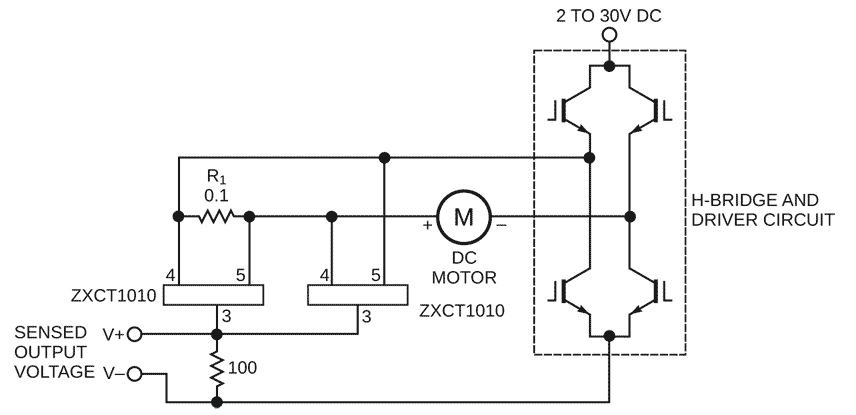# Circuit measures currents in dc servo motor

## Diodes ZXCT1010

The simple circuit design in Figure 1 lets you measure all components of a current flowing in a dc servo motor. The rectified output of the circuit uses ground as a reference, so you can measure the output by using a single-ended A/D converter. The current-sense resistor, R1, has a value of 0.1 Ω. The ZXCT1010 IC converts the differential signal across R1 to a single-ended signal. Two of these ICs form a signal rectifier. The single-ended signal makes measurement by an A/D converter cost-effective, small, and frugal in power consumption. The method also makes it possible to measure current from many sources at a time, such as in robots that use multiple servo motors. Measurement accuracy is approximately ±3%, which is adequate in most dynamic systems. Hence, an 8-bit A/D converter suffices to digitize the signal. If an average value of the current is of interest, then you can place an averaging capacitor between the V+ and V– terminals to remove the ac component. The unfiltered signal has 300-kHz response to ac current.Figure 1. With this simple circuit, you can measure the currents in a dc servo motor.

## Materials on the topic

EDN